﻿ Three Dimensional Face Surfaces Analysis using Geodesic Distance

### Three Dimensional Face Surfaces Analysis using Geodesic Distance

Rachid AHDID, Said SAFI, Bouzid MANAUT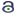OPEN ACCESS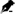PEER-REVIEWED

## Three Dimensional Face Surfaces Analysis using Geodesic Distance

Rachid AHDID1,, Said SAFI1, Bouzid MANAUT1

1Interdisciplinary Laboratory of Research in Sciences and Technologies (LIRST), Sultan Moulay Slimane University, Beni Mellal, Morocco

### Abstract

In this paper, we present an automatic 3D face recognition system based on the computation of the geodesic distance between the reference point and the other points in the 3D face surface. To compute a geodesic distance, we use the Fast Marching algorithm for solving the Eikonal equation. For space reduction, we apply Principal Component Analysis (PCA) and Fisher Linear Discriminant Analysis (LDA). Quantitative measures of similarity are obtained and then used as inputs to several classification methods. In the classifying step, we use: Neural Networks (NN), k-Nearest Neighbor (KNN) and Support Vector Machines (SVM). To test this method and evaluate its performance, a simulation series of experiments were performed on 3D Shape REtrieval Contest 2008 database (SHREC2008).

### At a glance: Figures

12
Prev Next

• AHDID, Rachid, Said SAFI, and Bouzid MANAUT. "Three Dimensional Face Surfaces Analysis using Geodesic Distance." Journal of Computer Sciences and Applications 3.3 (2015): 67-72.
• AHDID, R. , SAFI, S. , & MANAUT, B. (2015). Three Dimensional Face Surfaces Analysis using Geodesic Distance. Journal of Computer Sciences and Applications, 3(3), 67-72.
• AHDID, Rachid, Said SAFI, and Bouzid MANAUT. "Three Dimensional Face Surfaces Analysis using Geodesic Distance." Journal of Computer Sciences and Applications 3, no. 3 (2015): 67-72.

 Import into BibTeX Import into EndNote Import into RefMan Import into RefWorks

### 1. Introduction

The task of recognizing human face with the help of a machine has been has attracted more attention in recent years. Biometric face recognition technology has received significant attention in the past several years due to its potential in different applications. Automated human face recognition was applied in different fields including automated secured access to machines and buildings, automatic surveillance, forensic analysis, fast retrieval of records from databases in police departments, automatic identification of patients in hospitals, checking for fraud or identity theft, and human-computer interaction .

In a face recognition system, the individual is subject to a varied contrast and brightness lighting background. This three-dimensional shape when it is part of a two-dimensional surface as is the case of an image can lead to significant variations . The human face is an object of three-dimensional nature. This object may be subject of various rotations, not only flat but also space and also subject to deformations due to facial expressions. The shape and characteristics of this object also change over time .

Automatic face recognition based on the 2D images processing is well developed this last years, and several techniques have been proposed . There are a methods of 3D face recognition based on the use of three-dimensional information of the human face in the 3D space. Existing approaches that address the problem of 3D face recognition can be classified into several categories of approaches: Geometric or Local approaches 3D, Bronstein et al [1, 2] propose a new representation based on the isometric nature of the facial surface, Samir et al [3, 4] use 2D and 3D facial curves for analyzing the facial surface; Holistic approaches, Heseltine et al  have developed two approaches to applying the representations PCA Three-dimensional face, Cook et al  present a robust method for facial expressions based on Log-Gabor models from images of deep and some approaches are based on face segmentation can be found in [7-12].

The objective of this paper is to perform an automatic 3D face recognition system based on geodesic distance computing using Eikonal equation. For this we take the following steps:

•  Detection of 3D face where the nose end is a reference point.

•  Compute of geodetic distance between the reference point and the other points of the 3D facial surface using the Fast Marching algorithm as a solution of the Eikonal equation.

•  Reduction of geodesic distances space matrices by Principal Component Analysis (PCA) and Linear Discriminant Analysis (LDA) algorithms.

The rest of this paper is organized as follows: Section 2 describes the methodology of the proposed method with its stages: reference point detection, geodesic distance computing, Principal Component Analysis (PCA), Fisher Linear Discriminant Analysis (LDA) and classification algorithms (NN, KPPV and SVM). Section 3 includes the simulation results and method analysis. Section 4 draws the conclusion of this work and possible points for future work.

### 2. Methodology

Given an image of 3D face Shape REtrieval Contest 2008 database (SHRED2008) database our goal is to realize an automatic 3D face recognition system based on the computation of the geodesic distance between the reference point (nose tip) and the other points in the 3D face surface. So, our algorithm is divided to four main steps, first: Reference Point Detection, In this work we have detected the point of reference (nose tip) manually. Second: Geodesic Distance computation, an effective method to compute a geodesic distance between two points of facial surface is using the Fast Marching as a numerical algorithm for solving the Eikonal equation. Third: Dimensionality Reduction, in this step we use two known algorithms: Principal Component Analysis (PCA) and Fisher Linear Discriminant Analysis (LDA). Finally: classification algorithms, in this step we use three types of classification algorithms: the Neural Networks (NN), k-Nearest Neighbor (KNN) and Support Vector Machines (SVM). Figure (1) illustrates the steps of proposed method in our 3D face recognition system.

2.1. Reference Point Detection

The reference point (nose tip) is detected manually or automatically. There are several automatic approaches: L. Ballihi et al are developed an automatic algorithm nose end detection of a 3D face in . This algorithm is based on two cuts of facial surface. The first is the transverse face of the mass center. The second cut is based on the minimum depth point of the horizontal curve obtained by the first cut. The output of the last cut is a vertical curve and the minimum depth of this curve is the end of 3D face nose. In  S. Bahanbin et al use Gabor filters to automatically detect the nose tip. Another method has been used by C. Xu et al in , this method computes the effective energy of each neighbor pixel, then be determined the mean and variance of each neighbor pixel and uses the SVM to specify point end of the nose. L.H. Anuar et al  use a local geometry curvature and point signature to detect a nose tip region in 3D face model. In this work we have detected the point of reference p0 (nose tip) manually. The following figure (Figure 2) summarizes the steps to detect the nose tip of a 3D face of an image of the database SHREC2008.

Figure 2. 3D face nose end detection steps: (a) 3D face image; (b) Manual nose tip selection; (c) Reference point detection
2.2. Geodesic Distance

The geodesic distance between two points’ p0 and p of 3D face surface is the shortest path between the two points while remaining on the facial surface. In the context of calculating the geodesic distance R. Kimmel and J.A. Sethian  propose the method of Fast Marching as a solution of the Eikonal equation.

Eikonal equation is of the form:(1)

With:is an open set in Rn housebroken limit.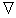denote the gradient.is the Euclidean norm.

The Fast Marching method is a numerical method for solving boundary value problems of the Eikonal equation [17, 18, 19]. The algorithm is similar to the Dijkstra's algorithm  and uses that information flows only to the outside from the planting area.

We consider a 3D face surface discretized using a triangular mesh with N vertices. Figure 3 shows a 3D face image of the Shape REtrieval Contest 2008 (SHREC2008) database whose obverse surface is discretized into a triangular mesh.

The geodesic distance between two points on a surface is calculated as the length of the shortest path connecting the two points. Using the Fast Marching algorithm on the triangulated surface 3D face, we can compute the geodesic distance between the reference point P0 and the other point’s p constructing the facial surface.

The geodesic distancebetween p0 and p is approximated by the following expression:(2)

With:

•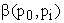is the path between p0 and according to the surface S of the 3D face.

•is the path length.

The following figure (Figure 4) shows the steps for determining the geodesic distance using a 3D face image of SHREC2008 database.

Figure 4. 3D face geodesic distance computes Steps: (a) 3D face image; (b) Reference point detection; (c) Discretization by triangular mesh; (d) Geodesic distance computing

Repeating this computation (geodesic distance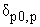) between the reference point p0 and each point p of the surface S of the 3D face, then we compute a geodesic distance matrix Ψ:With, δij =.

To realize our 3D face recognition system, we use the Principal Component Analysis (PCA) and Linear Discriminant Analysis (LDA) for writing space of the geodesic distance matrix [Ψ].

2.3. Principal Component Analysis (PCA)

The Principal Component Analysis (PCA) is to express a set of variables into a set of linear combinations of uncorrelated factors together, these factors account for a fraction of increasingly lower variability of the data. This method is used to represent the original data in a space of dimension less than the original space while minimizing the loss of information.

The recognition is performed by comparing the projection coefficients of a test image with those in the electronic driving components. The performance of the PCA will be illustrated by the following stages:

•  We load the data to be compared in a matrix Ψ.

•  We thus determine the size of the data set:(3)

- To summarize the data, we compute the sample mean vector and the sample standard deviation vector:(4)

•  Normalize the data. Here, the calibration means subtracting the average sample of each observation, and dividing by their standard deviation. This center and measure the data. Sometimes there are good reasons to change or do not perform this step, but we recommend you normalizes unless you have a good reason. It is easy to run this step as follows:(5)

•  The data matrix Ψ is multiplied by its transpose to obtain a covariance matrix L as shown in equation (5):(6)

•  In this step the values is computed using Matlab languages and the corresponding eigenvectors in the covariance matrix by the equation (6):(7)

Where V is an orthogonal matrix of specific vectors and D is a diagonal matrix of eigenvalues.

We classify the eigenvectors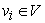according to decreasing values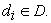The eigenvectors matrix V represents the projection eigenspace.

•  In this step we project the images vectors centered in eigenspace. For this, we must compute the scalar product of these pictures vectors along with the eigenvector matrix by:(8)
2.4. Equations Fisher Linear Discriminant Analysis (LDA)

The Linear Discriminant Analysis (LDA) of Fisher method proceeds according to the following steps:

2.4.1. Computing of within-class Scatter Matrix Sw

The within-class scatters matrix measure the amount of the dispersion between the images in the same class. For the i-th class, the dispersion matrix Si is calculated as the sum of the covariance matrices of the images centered in this category: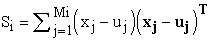(9)

Where μj is the mean of images in the class and xj is vector image. The matrix of the within-class dispersion Sw is the sum of all the dispersion matrices.(10)

Where c is the class’s number.

2.4.2. Computing of between-class Scatter Matrix Sb

As with tables and equations, figures should be set in one column if possible unless two-column display is essential. The resolution of graphics and image should be adequate to reveal the important detail in the figure.

Sb between-class scatter matrix measures the amount of dispersion between classes. It computes the sum of the difference between the total average and the average of each class.(11)

Where μi is the mean of images in class i and μ is the mean of all images.

2.4.3. Solve the Generalized Eigenvalue Problem

We calculate the eigenvalues and corresponding eigenvectors for the two dispersion matrices within-class and between-class by the following equation:(12)

Where Uk is a matrix of eigenvectors and λk is a matrix of eigenvalues.

The eigenvectors (each vector has the size of 1x100) are ordered in descending order of their eigenvalues. Finally we keep only the first C-1 eigenvectors. These C-1 eigenvectors form the Fischer projection base.

2.5. Classification

Let us consider the 3D human face images. First, we compute the geodesic distances matrix [Ψ] = δij as described in subsection (2.2). Then, we apply the reduce space algorithms (ACP or ALD) to geodesic distances matrix [Ψ], we obtain a low size matrix [A] = Aij. In the end, we determine the input vectors νi of classification algorithms using the linearization of the matrix [A]. Figure 5 illustrates the steps of extracting the input vectors of the classifier algorithms used in our face recognition system.

Figure 5. Steps of extracting input vectors of the classifier algorithms

To realize our 3D face recognition system, we use three types of classification algorithms: the Neural Networks (NN), k-Nearest Neighbor (KNN) and Support Vector Machines (SVM).

### 3. Simulation Results

In this section we make a series of simulation to evaluate the effectiveness of the proposed approach. These results were performed based on SHREC 2008 database. This database contain 427 scans of 61 subjects, for each of these 61 subjects 7 different scans namely: two “frontal”, one “look-up”, one “look-down”, one “smile”, one “laugh” and one “random expression” [21, 22].

The following table summarizes the results obtained from simulation:

#### Table 1. Resultants of Recognition rate and Error rate of our automatic 3D face recognition system

Table 1 summarizes the simulation results obtained by the presented approach. This results shows the recognition rate and the error rate found by methods used in our 3D face recognition system: (Geodesic Distance + PCA) and (Geodesic Distance + LDA) for characters extraction, with three algorithms used in classification such as: Neural Networks (NN), k-Nearest Neighbor (KNN) and Support Vector Machines (SVM).

The results obtained by our 3D face recognition system shows that our method present the best recognition rate (95,30%) using (Geodesic Distance + PCA) for images of SHREC 2008 database.

In conclusion of this series of results, a summary table (Table 2) compares the performance of our face authentication with respect to the performance obtained in other 3D face recognition systems.

#### Table 2. Comparison of Our Approach with Other Methods

We can notice that the performance of our automatic 3D face recognition system, In addition our system is perfect in all assessment. Our goal was to improve 3D faces recognition system we affirm based on the results that our goal is achieved.

### 4. Conclusion

In this paper, we have presented a 3D face recognition system based on the computation of the geodesic distance between the reference point and the other points in the 3D face surface. For writing space we have used PCA and LDA. For the classifying step we have implemented algorithms as NN, KNN and SVM. Simulation results give us a better recognition rate (95.3%) for the (ACP + DG + SVM) method. In future work, we will take a 3D face recognition system by analyzing of iso-geodesic curves using Riemannian geometry and compare these results with those obtained by this method.

### References

  Bronstein A. M., Bronstein M. M., Kimmel R. : Threedimensional face recognition. International Journal of Computer Vision 64, 1, 5-30. (2005).In article CrossRef  Bronstein A. M., Bronstein M. M., Kimmel R. : Expression-invariant representations of faces. IEEE Transactions on Image Processing Vol:16, Issue: 1 16, 188-197. (2007).In article  Samir C., Srivastava A., Daoudi M. : Three-dimensional face recognition using shapes of facial curves. IEEE Transactions on Pattern Analysis and Machine Intelligence, 1847-1857, (2006).In article CrossRef  Samir C., Srivastava A., Daoudi M., Klassen E. : An intrinsic framework for analysis of facial surfaces. International Journal of Computer Vision 82, 1, 80-95, (2009).In article CrossRef  Heseltine T., Pears N., Austin J. : Three-dimensional face recognition : an eigensurface approach. In ICIP, pp. 1421-1424. (Cité page 26.) (2004).In article CrossRef  Cook J. A., Chandran V., Fookes C. B. : 3d face recognition using log-gabor templates. In British Machine Vision Conference (Edinborugh, Scotland, 2006).In article CrossRef  Yan P., Bowyer K.W. : Biometric recognition using 3D ear shape. IEEE Transactions on Pattern Analysis and Machine Intelligence 29, 8, pp. 1297-1308. (2007).In article CrossRef PubMed  Chen H., Bhanu B. : Human ear recognition in 3D. IEEE Transactions on Pattern Analysis and Machine Intelligence 29, 4, pp. 718-737. (2007).In article CrossRef PubMed  Chang K. I., Bowyer K. W., Flynn P. J. : Multiple nose region matching for 3D face recognition under varying facial expression. IEEE Transactions on Pattern Analysis and Machine Intelligence 28, 10 (2006), pp. 1695-1700, (2006).In article  Drira H., Amor B. B., Srivastava A., Daoudi M. : A riemannian analysis of 3D nose shapes for partial human biometrics. In IEEE International Conference on Computer Vision, pp. 2050-2057, (2009).In article CrossRef  C.-S. Chua, F. Han and Y.-K. Ho : 3D human face recognition using point signature. In Proceedings of the Fourth IEEE International Conference on Automatic Face and Gesture Recognition 2000 (Washington, DC, USA, 2000), FG ’00, IEEE Computer Society, pp. 233-241, (2000).In article  T. C. Faltemier, K. W. Bowyer, P. J. Flynn: A region ensemble for 3-d face recognition. IEEE Transactions on Information Forensics and Security 3, 1,pp. 62-73 (2008).In article CrossRef  L. Ballihi, B. Ben Amor, M. Daoudi, A. Srivastava, D. Aboutajdine: Sélection de courbes de la surface nasale pour l’authentification de personnes en utilisant Adaboost. hal-00666262, version 1-3 Feb 2012.In article  S. Jahanbin, A. C. Bovik and H. Choi: Automated Facial Feature Detection from Portrait and Range Images. Image Analysis and Interpretation, 2008. SSIAI 2008. IEEE Southwest Symposium on, pp. 25 - 28, 24-26 March 2008.In article  C. Xu, Y. Wang, T. Tan, L. Quan: Robust nose detection in 3D facial data using local characteristics. Image Processing, ICIP '04. 2004 International Conference on (Volume: 3 ), pp. 1995-1998 (2004).In article  L.H. Anuar, S. Mashohor, M. Mokhtar and W.A. Wan Adnan: Nose Tip Region Detection in 3D Facial Model across Large Pose Variation and Facial Expression. IJCSI International Journal of Computer Science Issues, Vol. 7, Issue 4, No 4, July 2010.In article  R. Kimmel and J. A. Sethian, “Computing geodesic on manifolds,” in Proc. US National Academy of Science, 1998, vol. 95, pp. 8431-8435.In article PubMed  X. Desquesnes, A. Elmoataz and O. Lézoray : Eikonal equation adaptation on weighted graphs: fast geometric diffusion process for local and non -local image and data processing. Journal of Mathematical Imaging and Vision 46, 2 (2013), pp. 238-257, 2014.In article  E. Carlini, M. Falcone, N. Forcadel, R. Monneau: Convergence of a generalized fast-marching method for an eikonal equation with a velocity-changing sign. SIAM J. Numer. Anal. 46, 2920- 2952 (2008).In article CrossRef  E.W. Dijkstra, A Note on Two Problems in Connection with Graphs, Numerische Mathematik, 1 (1959), pp. 269-271, 1959.In article  Frank B. ter Haar, Mohamed Daoudi and Remco C. Veltkamp: SHape REtrieval Contest 2008: 3D Face Scans. Shape Modeling and Applications, 2008. SMI 2008. IEEE International Conference on, 4-6 June 2008.In article  Brian Amberg, Reinhard Knothe and Thomas Vetter: SHREC’08 Entry: Shape Based Face Recognition with a Morphable Model. Shape Modeling and Applications, 2008. SMI 2008. IEEE International Conference on, 4-6 June 2008.In article  C. Samir, M. Daoudi and A. Srivastava. A Framework of Calculus on Facial Surfaces. In 14th International Conference of Image Analysis andProcessing - Workshops, ICIAPW, 2007.In article  S. Feng, H. Krim, and I.A Kogan. 3D face recognition using euclidean integral invariants signature. In SSP ’07: IEEE/SP 14th Workshop on Statistical Signal Processing,, 156-160, Madison, WI, USA, 2007.In article  M. Daoudi, L. Ballihi, C. Samir, A. Srivastava. Tree-Dimensional Face Recognition Using Elastic Deformation Of Facial Surfaces. In IEEE, ICME, 2008.In article  T. Haar, F. B. and R.C. Veltkamp. SHREC’08 entry: 3D face recognition using facial contour curves. In SMI ’08: Proceedings of the IEEE International Conference on Shape Modeling and Applications, 259-260, Stony Brook, NY, USA, 2008.In article  A.S Gawali and P. R. R Deshmukh: 3D Face Recognition Using Geodesic Facial Curves to Handle Expression, Occlusion and Pose Variations. (IJCSIT) International Journal of Computer Science and Information Technologies, Vol. 5 (3), 4284-4287. 2014.In article  K. W. Bowyer, K. Chang and P. Flynn: A survey of approaches and challenges in 3D and multi-modal 3D + 2D face recognition. Computer Vision and Image Understanding, Elsevier. Volume 101, Issue 1, January 2006, Pages 1-15, 2006.In article  K. Lin, W. Cheng and J. Li: Facial Expression Recognition Based on Geometric Features and Geodesic Distance. International Journal of Signal Processing, Image Processing and Pattern Recognition. Vol.7, No.1, pp.323-330, 2014.In article  L.Y. Chong, A. B. Jin Teoh, T. S. Ong and S. C. Chong: 2,5D Face Recognition under Tensor Manifold Metrics. Springer International Publishing. Neural Information Processing. Lecture Notes in Computer Science Volume 8836, pp 653-660, 2014.In article CrossRef  R. Ahdid, S. Safi and B. Manaut: Approach of Facial Surfaces by Contour. IEEE Xplore, International Conference Multimedia Computing and Systems (ICMCS), DOI: 10.1109/ICMCS. 2014.6911284, p p: 465-468, 2014.In article